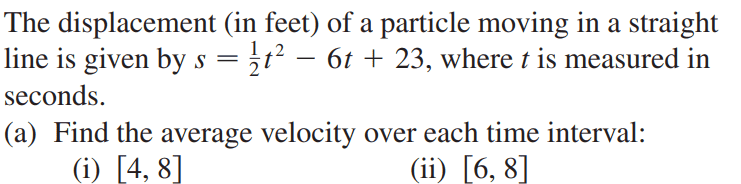# Average velocity of a particle

• ChiralSuperfields
In summary, Halc used the equation ##v_{avg} = \frac{1}{2}\Delta t - 6## to solve for the average velocity. However, he got -4 ft/s.f

#### ChiralSuperfields

Homework Statement
Relevant Equations
For this,The solution to (a)(i) is 0 ft/s. However I got -4 ft/s.

The formula I used was,
##v_{avg} = \frac{s_f - s_i}{t_f - t_i}##
##v_{avg} = \frac{\frac{1}{2}t^2_f - 6t_f + 23 - \frac{1}{2}t^2_i - 6t_i + 23}{t_f - t_i}##
##v_{avg} = \frac{ \frac{1}{2}(t^2_f - t^2_i) - 6(t_f - t_i)}{t_f - t_i}##

If someone could please point out what I did wrong, that would be much appreciated.

Many thanks!

Can't you just plug in the times in question to get the two positions in question? All the algebra seems unnecessary.

That aside, I don't see where the -4 comes from. Your bottom line seems correct.

Last edited:
•FactChecker, ChiralSuperfields and malawi_glenn
Can't you just plug in the times in question to get the two positions in question? All the algebra seems unnecessary.

That aside, I don't see where the -4 comes from. Your bottom line seems correct.

Sorry, my mistake. The formula that I forgot to write above was ## v_{avg} = \frac{1}{2}\Delta t - 6## because I mistakenly cancelled some of the change in times.

Many thanks!

1) You should include some parenthesis in your second line where you have substituted for ##s_i##.
The formula that I forgot to write above was ## v_{avg} = \frac{1}{2}\Delta t - 6##
2) This looks wrong to me. I think it should be ##v_{avg} = \frac{\frac{1}{2}(t_f+t_i)(t_f-t_i) - 6(t_f-t_i)}{(t_f-t_i)}= \frac{1}{2}(t_f+t_i) - 6##.
3) You could always follow the advice @Halc gave in post #2 of just calculating the initial and final positions. At least use that to check your algebra.

Last edited:
•ChiralSuperfields
##v_{avg} = \frac{ \frac{1}{2}(t^2_f - t^2_i) - 6(t_f - t_i)}{t_f - t_i}##

If someone could please point out what I did wrong, that would be much appreciated.
Formula seems correct. Did you simply forget the ##1\over 2## ?

 never mind•ChiralSuperfields
##v_{avg} = \frac{\frac{1}{2}t^2_f - 6t_f + 23 - \frac{1}{2}t^2_i - 6t_i + 23}{t_f - t_i}##
Typo: Missing parentheses around the ##t_i## terms, leading to wrong signs.
The formula that I forgot to write above was ## v_{avg} = \frac{1}{2}\Delta t - 6##
What is ##\frac{x^2-y^2}{x-y}##?

•ChiralSuperfields, FactChecker and jbriggs444
1) You should include some parenthesis in your second line where you have substituted for ##s_i##.

2) This looks wrong to me. I think it should be ##v_{avg} = \frac{\frac{1}{2}(t_f+t_i)(t_f-t_i) - 6(t_f-t_i)}{(t_f-t_i)}= \frac{1}{2}(t_f+t_i) - 6##.
3) You could always follow the advice @Halc gave in post #2 of just calculating the initial and final positions. At least use that to check your algebra.
 never mind